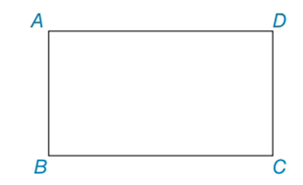Chapter 4.3, Problem 9EElementary Geometry For College St...

7th Edition
Alexander + 2 others
ISBN: 9781337614085

Solutions

Chapter
SectionElementary Geometry For College St...

7th Edition
Alexander + 2 others
ISBN: 9781337614085
Textbook Problem

In Exercises 8 to 10, use the properties of rectangles to solve each problem. Rectangle ABCD is shown in the figure.Exercises 8 - 10 Given: AB = 2x + 7 and BC = 3x + 4, and CD = 3x + 2 Find: x and DA

To determine

To find:

x and DA of the given rectangle.

Explanation

The basic property of rectangle:

1) All the properties of a parallelogram apply (the ones that matter here are parallel sides, opposite sides are congruent, and diagonals bisect each other).

2) All angles are right angles by definition.

3) The diagonals are congruent.

Calculation:

Given: AB = 2x + 7 and BC = 3x + 4, and CD = 3x + 2

Consider the rectangle.

Now we have to find x and DA of the given rectangle.

To find x:

From the property, opposite sides are congruent in rectangle.

So, AB = CD

2x+7=3x+23x

Still sussing out bartleby?

Check out a sample textbook solution.

See a sample solution

The Solution to Your Study Problems

Bartleby provides explanations to thousands of textbook problems written by our experts, many with advanced degrees!

Get Started

Test for divisibility by 2: 877,778

Elementary Technical Mathematics

2. Write each statement in exponential form.

Mathematical Applications for the Management, Life, and Social Sciences

In Exercises 4562, find the values of x that satisfy the inequality (inequalities). 54. x 4 1 and x + 3 2

Applied Calculus for the Managerial, Life, and Social Sciences: A Brief Approach

Using differentials, an approximation to (3.04)3 is: 28.094464 28.08 28.04 28

Study Guide for Stewart's Single Variable Calculus: Early Transcendentals, 8th